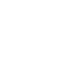BAIGROUPKZ-最舒适的旅行社

# 曼格斯托的吉普车之旅

1至5天

• 卡帕姆赛（峡谷）
• 夏克帕克神父（清真寺）
• 托里什（球状结节）
• 科卡拉（红山）
• 谢尔卡拉（山）
• 艾拉克蒂（山谷）

• 卡帕姆赛（峡谷）
• 夏克帕克神父（清真寺）
• 托里什（球状结节）
• 科卡拉（红山）
• 谢尔卡拉（山）
• 艾拉克蒂（山谷）

• 日加尔甘（堕落的土地）
• 苏丹伊普（清真寺）
• 卡帕姆赛（峡谷）

• 夏克帕克神父（清真寺）
• 布克蒂山
• 博兹拉（峡谷，上方3幅全景，从莱格（营地）底部下降）

• 博兹拉（照片区，轨道2公里）
• 克兹尔库普区域（红山，提拉米苏）
• 空心 卡拉吉
• 阿克套

• 托里什（球状结节）
• 谢尔卡拉
• 艾拉克蒂
• 图兹·拜尔（图兹拜尔）前3名全景照片下降到营地底部

• 图兹拜尔游览图兹拜尔（峡谷与盐湖）
• 纳伊萨（山）
• 博兹拉 （营地）

• 博兹拉游览
• 布克蒂山
• 克兹尔库普道
• 空心 卡拉吉
• 阿克套。

• 卡帕姆赛
• 夏克帕克神父
• 托里什
• 科卡拉
• 谢尔卡拉
• 艾拉克蒂（营地）

• 位置。 舍佩（商店，加油站）
• 图兹拜尔（顶部3幅全景图，下降到底部的营地）

• 图兹拜尔游览
• 奈扎
• 博兹拉 营地

• 博兹拉
• 布克蒂
• 克兹尔库普道
• 空心 卡拉吉
• 阿克套

• 索拉
• 日加尔甘
• 苏丹·埃佩
• 卡帕姆赛（营地）

• 夏克帕克神父
• 托里什
• 谢尔卡拉
• 科卡拉
• 艾拉克蒂（营地）

• 舍佩
• 图兹拜尔（营地）

• 图兹拜尔（游览）
• 奈扎
• 博兹拉（勒格）

• 博兹拉（游览）
• 布克蒂
• 克兹尔库普道
• 空心 卡拉吉
• 阿克套

• 卡帕姆赛
• 夏克帕克神父
• 托里什
• 科卡拉
• 谢尔卡拉
• 艾拉克蒂（营地）

• 位置。 舍佩（商店，加油站）
• 图兹拜尔（顶部3幅全景图，下降到底部的营地）

• 图兹拜尔游览
• 奈扎
• 博兹拉 营地

• 博兹拉
• 布克蒂
• 克兹尔库普道
• 费蒂索夫（里海海湾）
• 托卡马克（沿海岩石）
• 托卡马克开普（在海岸过夜）

• 托卡马克斗篷（游泳）
• 皮克库里克
• 阿克套

* 运输
* 指南翻译
* 餐点（早餐，晚餐，早餐，午餐）
* 露营设备（帐篷，靠垫，睡袋，床单）

+77473511779
+77273177557
info@baigroupkz.com
Республика Казахстан Алматы мкрн. Шапагат ул. 3-Градокомплекс 166